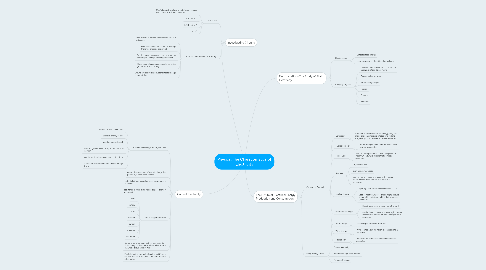# Physics: The Characteristics of Electricity

Get Started. It's FreePhysics: The Characteristics of Electricity## 1. Electrostatics - The Study of Static Electricity

### 1.1. Electroscope

1.1.1. Detects static charge

1.1.2. Leaves open when the object has a charge

### 1.2. Charging Objects

1.2.1. Only electrons move form one object to another, protons do not move

1.2.2. Opposite charges attract

1.2.3. Similar charges repel

1.2.4. Friction

1.2.5. Contact

1.2.6. Induction

## 2. Current Electricity

### 2.1. Current Electricity, Circuits, and Cells

2.1.1. Continuous flow of electrons

2.1.2. needs an energy source

2.1.3. needs a complete path

2.1.4. fuel cells generate electricity from a chemical reaction

2.1.5. load turns electrical charge into another form

2.1.6. a conductor allows electrons to flow through them

2.5.1. wire

2.5.2. battery

2.5.3. lamp

2.5.4. resistor

2.5.5. switch

2.5.6. ammeter

2.5.7. voltmeter

## 3. Investigating Circuits

### 3.1. Ohm's Law

3.1.1. Ohm's Law is the relationship between voltage and current in an ideal conductor.

3.1.2. V=1R unit is V

3.1.3. I=V/R unit is A

3.1.4. R=V/I

### 3.2. Short Circuits and Electrical Safety

3.2.1. Short circuits is caused by having too low of a resistance

3.2.2. Fuse melts when the current is too high therefore breaking the circuit

3.2.3. Circuit breaker heats up and bends when the current gets too high, breaking the circuit

3.2.4. Three-pong plug connects the device to the ground wire of a building

3.2.5. Ground fault circuit interrupter detects change in current flow

## 4. The Future of Electrical Energy Production and Consumption

### 4.1. Generating Electricity

4.1.1. Generators

4.1.1.1. transform motion into electric energy, magnets inside the generators are rotated by a turbine, magnets spin copper wire, wire creates current.

4.1.2. Hydroelectricity

4.1.2.1. Water from penstock turns a turbine which powers a generator

4.1.3. Fossil Fuels

4.1.3.1. Used to heat up water to create high pressure steam which turns a turbine that powers a generator

4.1.4. Biomass

4.1.4.1. Organic material

4.1.4.2. plant and animal waste

4.1.4.3. used to hear tup water to create high pressure steam which turns a turbine that powers a generator.

4.1.5. Nuclear Energy

4.1.5.1. Splitting of atoms of heavy elements occur

4.1.5.2. used to heat up water to create high pressure steam which rotates a turbine that powers a generator.

4.1.6. Geothermal Energy

4.1.6.1. Hated water making steam from the Earth

4.1.6.2. used to heat up water to create high pressure steam which rotates a turbine that powers a generator.

4.1.7. Solar Energy

4.1.7.1. Uses sunlight to collect electrons

4.1.8. Wind Energy

4.1.8.1. Wind rotates a turbine which is connected to a generator

4.1.9. Tidal Energy

4.1.9.1. Tides rotate a turbine which is connected to a generator

### 4.2. Being Energy Smart

4.2.1. Cost of electricity

4.2.2. Electrical energy consumption

4.2.3. Percent efficiency